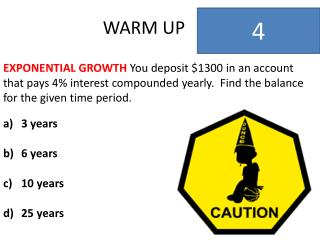DownloadDownload PresentationWARM UP

# WARM UP

Download Presentation## WARM UP

- - - - - - - - - - - - - - - - - - - - - - - - - - - E N D - - - - - - - - - - - - - - - - - - - - - - - - - - -
##### Presentation Transcript

1. WARM UP 4 EXPONENTIAL GROWTH You deposit \$1300 in an account that pays 4% interest compounded yearly. Find the balance for the given time period. 3 years 6 years 10 years 25 years

2. WARM UP 3 EXPONENTIAL GROWTH You deposit \$1300 in an account that pays 4% interest compounded yearly. Find the balance for the given time period. 3 years 6 years 10 years 25 years

3. WARM UP 2 EXPONENTIAL GROWTH You deposit \$1300 in an account that pays 4% interest compounded yearly. Find the balance for the given time period. 3 years 6 years 10 years 25 years

4. WARM UP 1 EXPONENTIAL GROWTH You deposit \$1300 in an account that pays 4% interest compounded yearly. Find the balance for the given time period. 3 years 6 years 10 years 25 years

5. WARM UP 0 EXPONENTIAL GROWTHYou deposit \$1300 in an account that pays 4% interest compounded yearly. Find the balance for the given time period. 3 years 6 years 10 years 25 years

6. 8.7 Exponential DecayFunctions GOAL Write exponential decay functions. KEY WORDS Exponential decay Decayrate Decayfactor

7. 8.7 Exponential Decay Functions EXONENTIAL DECAY A quantity is decreasing exponentially if it decreases by the same percent r in each unit of time t. This is called exponential decay. Exponential decay can be modeled by the equation y = C(1 - r)t

8. 8.7 Exponential Decay Functions EXONENTIAL DECAY y = C(1 + r)t C is the initial amount (the amount before any decayoccurs), r is the decayrate (as a decimal), t represents time. The expression (1 - r) is called the decayfactor.

9. 8.7 Exponential Decay Functions EXAMPLE 1 Write an Exponential DecayModel CARS You bought a car for \$16,000. You expect the car to lose value, or depreciate, at a rate of 12% per year. Write an exponential decay model to represent the situation.

10. 8.7 Exponential Decay Functions EXAMPLE 1 Write an Exponential Growth Model SOLUTION Let y be the value of the car and let t be the number of years of ownership. The initial value of the car C is \$16,000. The decay rate r is 12%, or 0.12. y = C(1 - r)t = 16,000(1 - 0.12)t = 16,000(0.88)t

11. 8.7 Exponential Decay Functions Checkpoint Write an Exponential DecayModel 1. Your friend bough a car for \$24,000. The car depreciates at the rate of 10% per year. Write an exponential model to represent the car’s value.

12. 8.7 Exponential Decay Functions EXAMPLE 2 Use the Exponential Decay Model Use the model in Example 1 to find the value of your car after 8 years.

13. 8.7 Exponential Decay Functions EXAMPLE 2Use the Exponential Decay Model SOLUTION To find the value after 8 years, substitute for t. A = P(1 + r)t = 16,000(0.88)t = 500(0.88)8 =5754

14. 8.7 Exponential Decay Functions Checkpoint Use an Exponential Decay Model Use the model in Checkpoint 1. 2. Find the value of your friend’s car after 6 years.